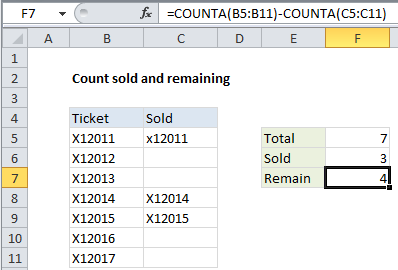## Excel Office

Excel How Tos, Tutorials, Tips & Tricks, Shortcuts

# Count sold and remaining in Excel

This tutorial shows how to Count sold and remaining in Excel using the example below;

## Formula

`=COUNTA(range1)-COUNTA(range2)`## Explanation

If you have a list of items, and need to count how many you have total, how many are sold, how remain, etc., you can use the COUNTA function. This can be useful if you are selling tickets, seats, entries, or anything where you maintain and track an inventory of items sold.

Worked Example:   How to create dynamic named range with INDEX in Excel

In the example, the formula in F7 is:

`=COUNTA(B5:B11)-COUNTA(C5:C11)`

The COUNTA function counts non-blank cells that contain numbers or text. The first COUNTA counts non-blank cells in the range B5:B11 and returns the number 7:

`COUNTA(B5:B11) // returns 7`

The second COUNTA function does the same with the range C5:C11 and returns 3, since there are 3 non-blank cells in that range:

`COUNTA(C5:C11) // returns 3`

So, the entire formula is reduced to 7 – 3 and returns 4.

Worked Example:   Highlight duplicate columns in Excel

Note that in this case the values that appear in column C don’t matter. They could be the the codes from column B (as in the example), the word “yes”, or simply “x”.

Worked Example:   How to return TRUE when all cells in range are blank in Excel

### Match test

If you need to make sure that the value in column C matches the value in column B, in the same row, you can use a formula based on SUMPRODUCT instead:

`=SUMPRODUCT(--(B5:B11=C5:C11))`